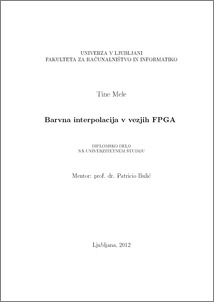# FPGA based color interpolation

Tine Mele (2012) FPGA based color interpolation. EngD thesis.Preview
PDF

## Abstract

The purpose of this thesis is the implementation of the FPGA (Field Programmable Gate Array) core, that converts picture from Bayer pattern to full colored image. We used CMOS (Complementary Metal Oxide Semiconductor) image sensor with 2048x1088 pixel resolution and 340 fps (frames per second) frame rate. A Bayer pattern presents picture with only one color for each pixel. A type of Bayer filter determines if the color on selected pixel is red, green or blue. The remaining two color values need to be calculated. Four algorithms for calculation of missing colors are described in this thesis. These algorithms are bilinear interpolation, nearest neighbour interpolation, Cok algorithm and linear interpolation with s filter size of $5\times5$ pixels. One of those is selected and implemented on the FPGA logic circuit. To make the right choice, we have to measure the quality of the images that we get from each algorithms. For this purpose we present three methods for evaluation of image quality. Before the final selection, we compare the number of required elements in the FPGA logic circuit, according to the algorithm that we want to use. We developed architecture for each algorithm and on this basis, we compare the required number of logic gates. Since the exact number can not be determined in advance, we estimate by comparing the required number of adders, countdowns, multipliers, dividers and memory size for the implementation of each algorithm. Based on the assessment of image quality and number of required elements on the FPGA circuit, we made a conclusion that the most appropriate algorithm for the implementation is linear interpolation with a filter size of 5x5 pixels. The implementation of this algorithm is described in detail at the end.

Item Type: Thesis (EngD thesis)
Keywords: FPGA, CMOS sensor, Bayer filter, color interpolation
Number of Pages: 69
Language of Content: Slovenian
Mentor / Comentors:
Name and SurnameIDFunction
prof. dr. Patricio Bulić255Mentor